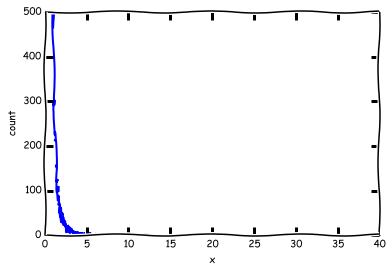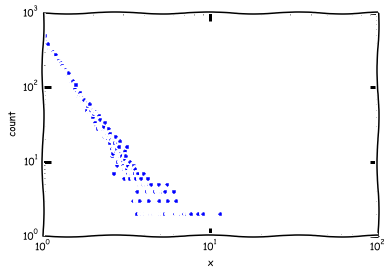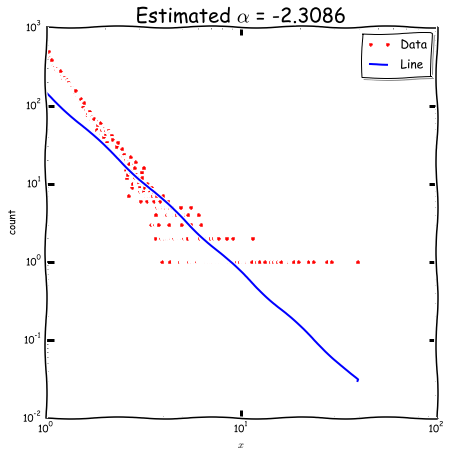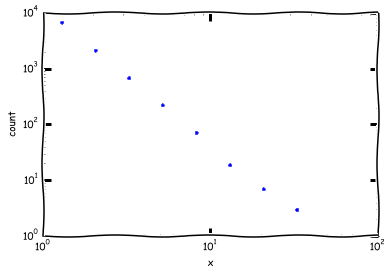# Power Law¶

In :
import numpy as np
import matplotlib.pyplot as plt
plt.xkcd()
import numpy.random as rnd
%matplotlib inline


### Generating Power Law Distribution¶

So, the pdf of the power law distribution is $$p(x) = Cx^{-\alpha}\text{, }$$ where $C$ is normalization constant and $\alpha>0$ is called as exponent of the distribution.

From the lecture we know that (unless seminar starts before. If it is so, let's derive...)$$C = \frac{\alpha - 1}{x_{\text{min}}^{- \alpha + 1}}.$$ Let's try to generate power law random variable (and pretend we don't know np.pareto() ).

EXERCISE 1

The first step is to derive cdf for powel law: $F(x) = P(X \leq x)$

$$F(x) = 1 - \int_{x}^\infty p(t) dt$$

(here some random student goes to the whiteboard...)

In :
numStud = 10
print 'Student {0:2.0f} is going to the whiteboard'.format(np.round(rnd.random()*(numStud-1) + 1))

Student  9 is going to the whiteboard


SOLUTION

$$F(x) = 1 - (\frac{x}{x_\text{min}})^{ - \alpha + 1}$$

EXERCISE 2

If we define a random variable $R$, s.t. $R = F(X)$, then $R$ will be uniformly distributed on interval [0, 1].

Good thing here is that we easily can generate uniformly distributed pseudorandom numbers. Let's find an expression for $x = F^{-1}(r)$.

SOLUTION

\begin{equation} x = (1 - r)^{\frac{-1}{\alpha - 1}} x_\text{min} \end{equation}

EXERCISE 3

1. Generate 10000 uniformly distributed pseudorandom numbers. Set alpha - 1 = 2.5 and x_min = 1
2. Produce power law!
3. Plot histogram of the results (instead of using plt.histogram() use np.histogram( ,bins = 5000 ))
4. See something unpleasant?
In :
# Write your code here..
#
#

# Generate uniform pseudorandom variables
r = rnd.random(10000)
alpha = 3.5
xmin = 1.0;

# Get power law!!!
x = (1 - r)**(-1.0/(alpha - 1)) * xmin

In :
# Plot histogramm

yh, binEdges=np.histogram(x, bins=2000)
bincenters = 0.5*(binEdges[1:]+binEdges[:-1])
plt.plot(bincenters, yh, '-', lw=2)
plt.ylabel('count')
plt.xlabel('x')

Out:
<matplotlib.text.Text at 0xa7f3048>In :
# That looks not good...
# Let's plot in log-log scale!

plt.loglog(bincenters, yh, '.', lw=2)
plt.ylabel('count')
plt.xlabel('x')

Out:
<matplotlib.text.Text at 0xa813128>## Exponent Estimation¶

EXERCISE 4

Given the results you've obtained in the previous section, try to estimate $\alpha$ via Linear Regression (don't forget to take $\log$)

In :
# Write your code here..
#
#

# Move zeros away!
idx = np.ix_(yh != 0)
x_est = np.log(bincenters[idx])
y_est = np.log(yh[idx])
s = len(x_est)

# Do estimation
X = np.vstack([np.ones(s), x_est]).T
Beta = np.dot(np.dot(np.linalg.inv(np.dot(X.T, X)), X.T), y_est)

# Plot
idx = np.argsort(x_est)
yt = np.exp(X[idx,:].dot(Beta))
xt = np.exp(X[idx,1])

fig, ax = plt.subplots()
fig.set_size_inches(7,7)
ax.loglog(bincenters, yh, '.r', label='Data')
ax.loglog(xt, yt, 'b', label='Line')
plt.ylabel('count')
plt.xlabel('$x$')
plt.title('Estimated $\\alpha$ = {0:1.4f}'.format(Beta), fontsize=20)

Out:
<matplotlib.text.Text at 0xb5c55f8>Probably, you won't be satisfied with the results.. Btw, why?

Thankfully, there are some options to solve the problem:

1. Exponential binning
2. CDF estimation
3. Likelyhood estimation



During the seminar we will work with 1 (and maybe 2).

EXERCISE 5

Perform exponential binning, that is, instead of using uniformal bins, spread them with log-scaling

hint: use use np.logspace()

In :
# Write your code here..
#
#

# Binning
bins = np.logspace(0, 6, base = 2.0, num = 10)
yh, binEdges = np.histogram(x, bins)
bincenters = 0.5*(binEdges[1:]+binEdges[:-1])

# Plot
plt.loglog(bincenters, yh, '.', lw=2)
plt.ylabel('count')
plt.xlabel('x')

# Move zeros away!
idx = np.ix_(yh != 0)
x_est = np.log(bincenters[idx])
y_est = np.log(yh[idx])
s = len(x_est)

# Do estimation
X = np.vstack([np.ones(s), x_est]).T
Beta = np.dot(np.dot(np.linalg.inv(np.dot(X.T, X)), X.T), y_est)
print Beta

[ 9.41162892 -2.43875212]